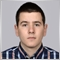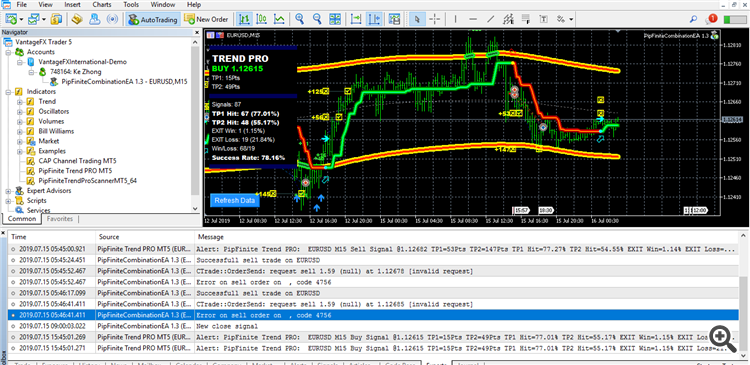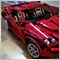# Invalid request / error number 4756 MQL5 EA5092

Hi guys,

I am using an EA to go through a few symbols in a list and also a few time frames, it worked good ,but lately i get a few errors for invalid request. I am using the CTrade library to open and manage the trades, and considering i dont work with the request struct directly i dont understand why does the error comes up. It comes up after I check that i got a successful trade. So basically the boolean that is used to check the return of the Ctrade position open, returns true, and after that the error comes.I suppose this image will help understand.

```void OrderEntry(string symbol,ENUM_TIMEFRAMES time,int direction)
{
double stoplevel=SymbolInfoInteger(symbol,SYMBOL_TRADE_STOPS_LEVEL)*point1;

int pts=0;
if(SymbolInfoDouble(symbol,SYMBOL_VOLUME_MIN)==0.01)pts=2;
if(SymbolInfoDouble(symbol,SYMBOL_VOLUME_MIN)==0.1)pts=1;
double tp=0,sl=0;
double Bid = SymbolInfoDouble(symbol,SYMBOL_BID);
double Ask = SymbolInfoDouble(symbol,SYMBOL_ASK);
double TP1 = GetLastTP1(symbol,time);
double TP2 = GetLastTP2(symbol,time);
if(direction==0)
{
if(TakeProfit3>0)
{
tp=NormalizeDouble((Bid+TakeProfit3*point1),digits1);
if(TakeProfit3*point1<stoplevel)tp=NormalizeDouble((Bid+stoplevel),digits1);
else{tp=0;}
}
if(TakeProfit2==On)
{
tp=TP2;
}
if(TakeProfit1==On)
{
tp=TP1;
}

if(StopLoss>0)
{
sl=NormalizeDouble(Bid-StopLoss*point1,digits1);
if(StopLoss*point1<stoplevel)sl=NormalizeDouble((Bid-stoplevel),digits1);
}
bool buyop=ExtTrade.PositionOpen(symbol,ORDER_TYPE_BUY,NormalizeLots(symbol,NewVolume(symbol,time)),Ask,sl,tp);
if(buyop)
{
Print("Successfull buy trade on ",symbol);
tkt_size++;
ArrayResize(tkt,tkt_size);
ArrayResize(trlevel,tkt_size);
trlevel[tkt_size-1]=Bid;
tkt[tkt_size-1]=LastTicket(symbol);
SendMail("Order Opened !","Success opening buy trade at "+DoubleToString(Ask)+" /SL "+DoubleToString(sl)
+" /TP "+DoubleToString(tp)+" on "+symbol);
}
else
{
Print("Error on buy order on ",symbol," , code ",GetLastError());
SendMail("Order Opened !","FAIL opening buy trade at "+DoubleToString(Ask)+" /SL "+DoubleToString(sl)
+" /TP "+DoubleToString(tp)+" on "+symbol);
}
}
if(direction==1)
{
if(TakeProfit3>0)
{
tp=NormalizeDouble((Ask-TakeProfit3*point1),digits1);
if(TakeProfit3*point1<stoplevel)tp=NormalizeDouble((Bid+stoplevel),digits1);
}else{tp=0;
}
if(TakeProfit2==On)
{
tp=TP2;
}
if(TakeProfit1==On)
{
tp=TP1;
}

if(StopLoss>0)
{
sl=NormalizeDouble((Ask+StopLoss*point1),digits1);
if(StopLoss*point1<stoplevel)sl=NormalizeDouble((Ask+stoplevel),digits1);
}
bool sellop=ExtTrade.PositionOpen(symbol,ORDER_TYPE_SELL,NormalizeLots(symbol,NewVolume(symbol,time)),Bid,sl,tp);
if(sellop)
{
Print("Successfull sell trade on ",symbol);
tkt_size++;
ArrayResize(tkt,tkt_size);
ArrayResize(trlevel,tkt_size);
trlevel[tkt_size-1]=Ask;
tkt[tkt_size-1]=LastTicket(symbol);
SendMail("Order Opened !","Success opening sell trade at "+DoubleToString(Bid)+" /SL "+DoubleToString(sl)
+" /TP "+DoubleToString(tp)+" on "+symbol);
}
else
{
Print("Error on sell order on ",symbol," , code ",GetLastError());
SendMail("Order Opened !","Fail opening sell trade at "+DoubleToString(Bid)+" /SL "+DoubleToString(sl)
+" /TP "+DoubleToString(tp)+" on "+symbol);
}
}
}```

ALso i sent the code used to open trades, hope you can help !931

Stanislav Ivanov:

Hi guys,

I am using an EA to go through a few symbols in a list and also a few time frames, it worked good ,but lately i get a few errors for invalid request. I am using the CTrade library to open and manage the trades, and considering i dont work with the request struct directly i dont understand why does the error comes up. It comes up after I check that i got a successful trade. So basically the boolean that is used to check the return of the Ctrade position open, returns true, and after that the error comes.

I suppose this image will help understand.

ALso i sent the code used to open trades, hope you can help !

From the log, looks like your OrderEntry() was given an empty 'symbol'. Will be useful if you can share how you declare your "few symbols in a list", and how you iterate it and make calls to OrderEntry().14129

Upd: No, the problem is different.14129

Try this
```      else
{
MqlTradeResult Result;
MqlTradeRequest Request;

ExtTrade.Request(Request);
ExtTrade.Result(Result);

Print(ToString(Request) + ToString(Result));

Print("Error on sell order on ",symbol," , code ",GetLastError());
SendMail("Order Opened !","Fail opening sell trade at "+DoubleToString(Bid)+" /SL "+DoubleToString(sl)
+" /TP "+DoubleToString(tp)+" on "+symbol);
}```

Форум по трейдингу, автоматическим торговым системам и тестированию торговых стратегий

ФОРТС. Вопросы по исполнению

fxsaber, 2018.02.28 13:39

```#define TOSTRING(A)  #A + " = " + (string)(A) + "\n"
#define TOSTRING2(A) #A + " = " + EnumToString(A) + " (" + (string)(A) + ")\n"

string ToString( const MqlTradeRequest &Request )
{
return(TOSTRING2(Request.action) + TOSTRING(Request.magic) + TOSTRING(Request.order) +
TOSTRING(Request.symbol) + TOSTRING(Request.volume) + TOSTRING(Request.price) +
TOSTRING(Request.stoplimit) + TOSTRING(Request.sl) +  TOSTRING(Request.tp) +
TOSTRING(Request.deviation) + TOSTRING2(Request.type) + TOSTRING2(Request.type_filling) +
TOSTRING2(Request.type_time) + TOSTRING(Request.expiration) + TOSTRING(Request.comment) +
TOSTRING(Request.position) + TOSTRING(Request.position_by));
}

string ToString( const MqlTradeResult &Result )
{
return(TOSTRING(Result.retcode) + TOSTRING(Result.deal) + TOSTRING(Result.order) +
TOSTRING(Result.volume) + TOSTRING(Result.price) + TOSTRING(Result.bid) +
TOSTRING(Result.ask) + TOSTRING(Result.comment) + TOSTRING(Result.request_id) +
TOSTRING(Result.retcode_external));
}```
To add comments, please log in or register